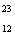/
/
/
Multiple Choice 1.Which statement incorrect? A.  According to the atomic theory, all
Not my Question
Flag Content

# Question : Multiple Choice 1.Which statement incorrect? A.  According to the atomic theory, all : 1409657

Multiple Choice

1.Which statement is incorrect?

A.  According to the atomic theory, all matter is composed of atoms.

B.  Protons, neutrons, and electrons are subatomic particles.

C.  Electrons have greater mass than protons.

D.  Neutrons are found in the nucleus of the atom.

2.Choose the incorrect statement about the proton:

A.  The proton has the atomic mass of 1 amu.

B.  The proton has the same charge as the neutron.

C.  The proton has greater mass than an electron.

D.  The proton and the neutron have approximately the same atomic mass.

3.The atom’s structure characteristically has

A.  the protons and neutron within the nucleus.

B.  the electrons located outside the nucleus.

C.  mostly empty space.

D.  All of these choices are correct.

4.Which of the substances listed below is not an element?

A.  sodium

B.  iron

C.  air

D.  carbon

5.  Atomic number and mass number differ in that atomic number is the number of _____ and mass number is _____ in an atom's nucleus.

A.  protons/the number of neutrons

B.  neutrons/the number of protons

C.  protons/the number of electrons

D.  protons/the sum of protons and neutrons

6.  An atom of cobalt with a mass number of 60 has how many neutrons?

A.  27

B.  33

C.  59

D.  60

7.  All atoms of the same element that have the same atomic number, but different mass numbers are known as  _____

A. Protons

B. moles

C. isomers

D. isotopes

8.The mass in grams of one mole of an element is the element's _____.

A.  atomic number

B.  atomic weight

C.  molar mass

D.  isotope number

9.Mass number refers to

A. total number of protons and electrons

B. total number of protons

C. total number of neutrons and protons

D. total number of neutrons and electrons

10.Mg has how many protons, neutrons, and electrons?

A.  12 protons, 23 neutrons, and 23 electrons

B.  12 protons, 11 neutrons, and 11 electrons

C.  23 protons, 11 neutrons, and 23 electrons

D.  12 protons, 11 neutrons, and 12 electrons

1

## Solution 5 (1 Ratings )

Solved
Chemistry 1 Year Ago 46 Views